# Tile Border

Mrs. Garcia wants to put a tile border along one side of her kitchen wall. Mrs. Garcia measures the wall and knows that she needs enough tiles to make three rows with sixty-three tiles in each row. How many tiles does Mrs. Garcia need to make the border? Tiles sell in boxes with eight tiles in each box. How many boxes of tiles does Mrs. Garcia need to buy? Write equations to support your mathematical thinking.

Assessment

## Plan

#### Formal Mathematical Language and Symbolic Notation

A student may independently select a printed number line, number chart, ten frames, graph paper, etc. as they work on a task.

### Suggested materials

Engagement Image:

Teachers may project the image below to launch this task for their students, define nouns, promote discussion, access prior knowledge, and inspire engagement and problem solving.

## Solutions tabs

### Expert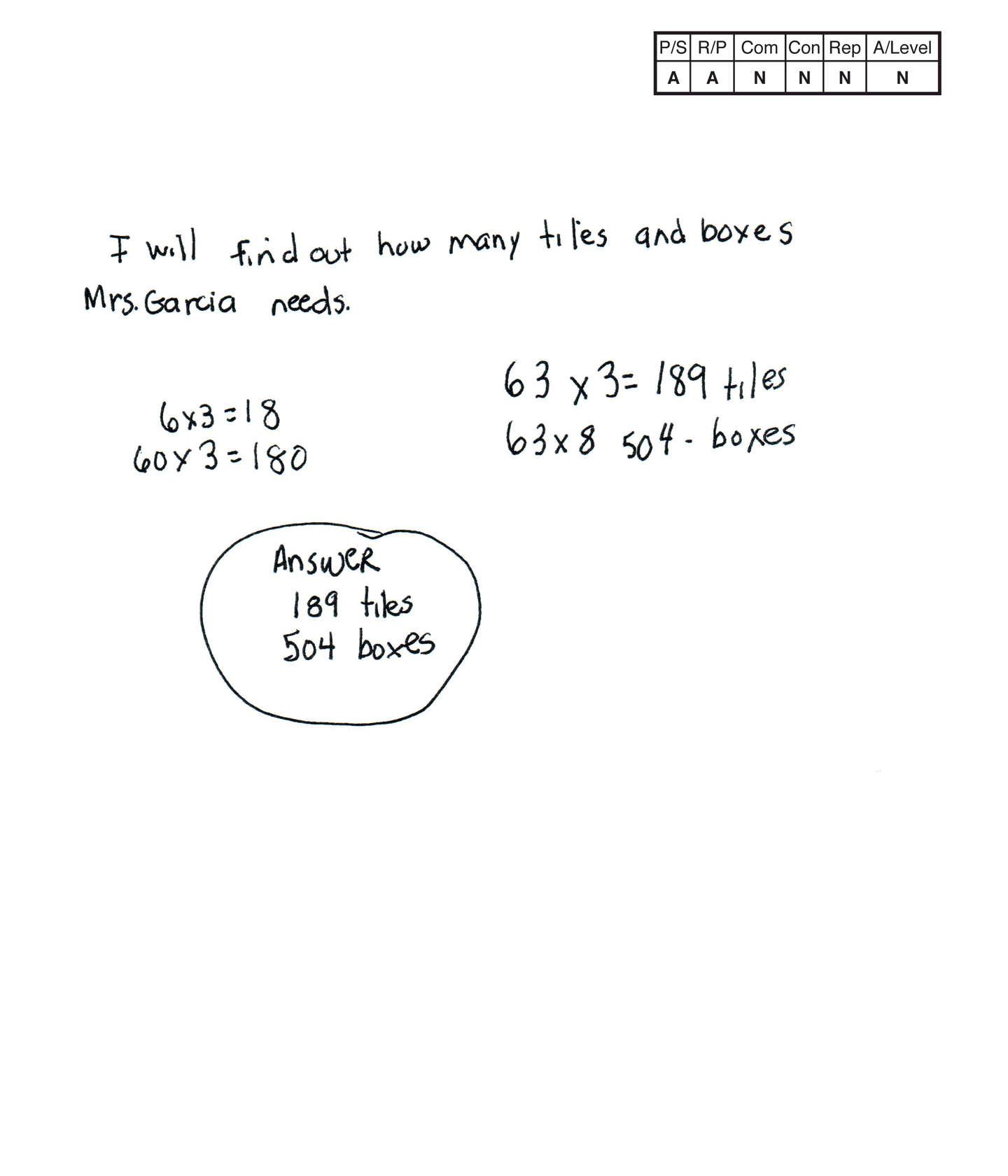This student does not meet the standard.

### Scoring Rationale

#### Apprentice

The student’s strategy to use multiplication to find the total number of tiles Mrs. Garcia needs works to solve the task. The student’s answer, “189 tiles,” is correct. The student is not able to solve the second part of the task correctly. The student’s answer, “504 boxes,” is not correct.

#### Apprentice

The student demonstrates correct reasoning for the first part of the task by applying a correct multiplication equation. The student does not show correct reasoning for the second part of the task. The student multiplies 63 tiles by 8 tiles instead of dividing the 189 total tiles by 8 tiles per box.

#### Novice

The student does not use any mathematical language or notation.

#### Novice

The student does not make a mathematically relevant connection.

#### Novice

The student does not use any mathematical representation in their solution.

#### Novice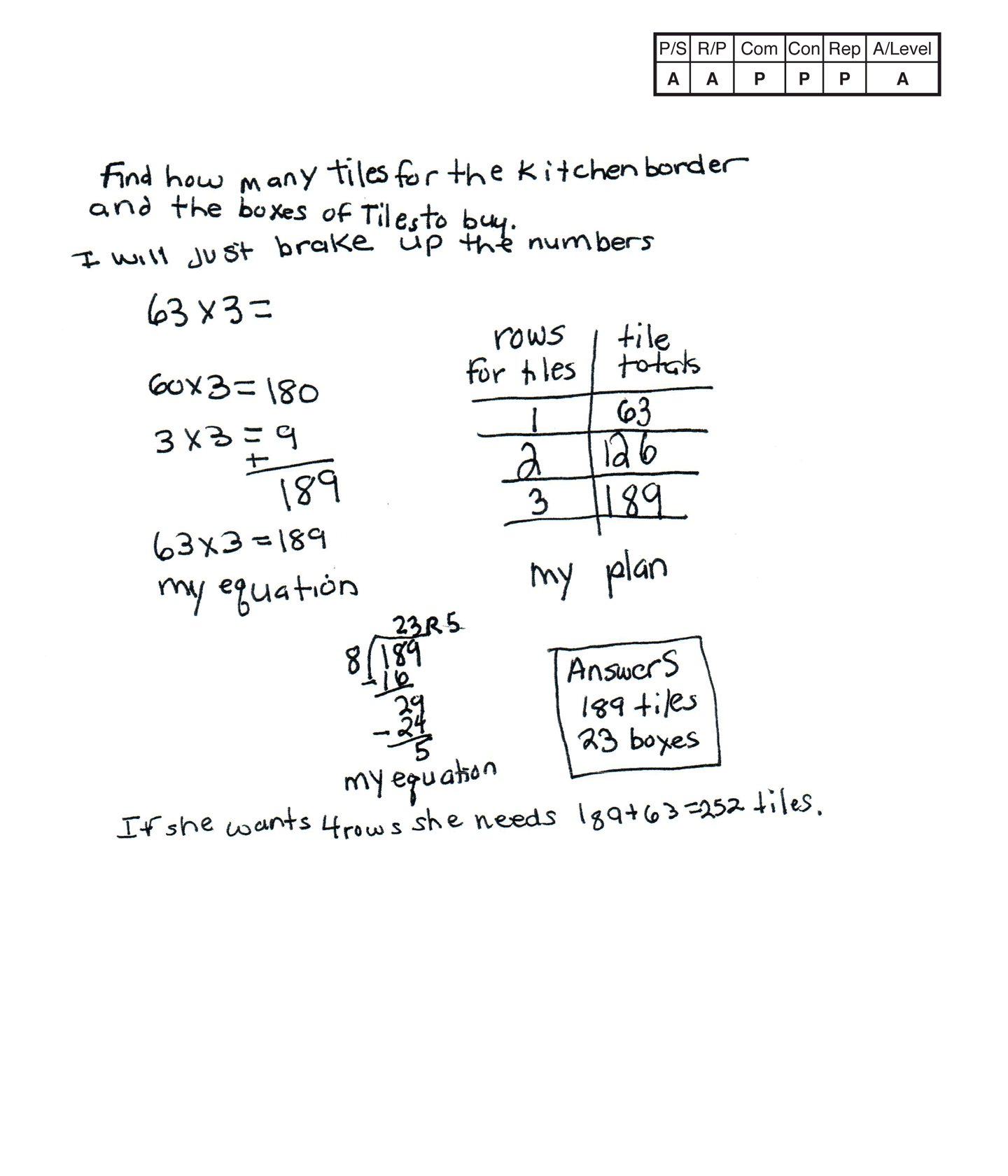This student does not meet the standard.

### Scoring Rationale

#### Apprentice

The student’s strategy to use multiplication and a table to find the total number of tiles Mrs. Garcia needs works to solve the task. The student’s answer, “189 tiles,” is correct. The student uses division to find the total number of boxes of tiles Mrs. Garcia needs. The student fails to consider the remainder of five tiles which results in an incorrect answer of “23 boxes.”

#### Apprentice

The student demonstrates correct reasoning for the first part of the task by applying a correct multiplication equation. The student does not show correct reasoning for the second part of the task. The student uses division correctly but does not realize that 24 boxes of tile are needed because of the 5 tile remainder when dividing 189 tiles by 8 tiles.

#### Practitioner

The student correctly uses the mathematical terms row, equation from the task. The student also correctly uses the term total.

#### Practitioner

The student makes the mathematically relevant observation, “If she wants 4 rows she needs 189 + 63 = 252 tiles.

#### Practitioner

The student’s table is appropriate and accurate. All labels are included and the data is correct.

#### Apprentice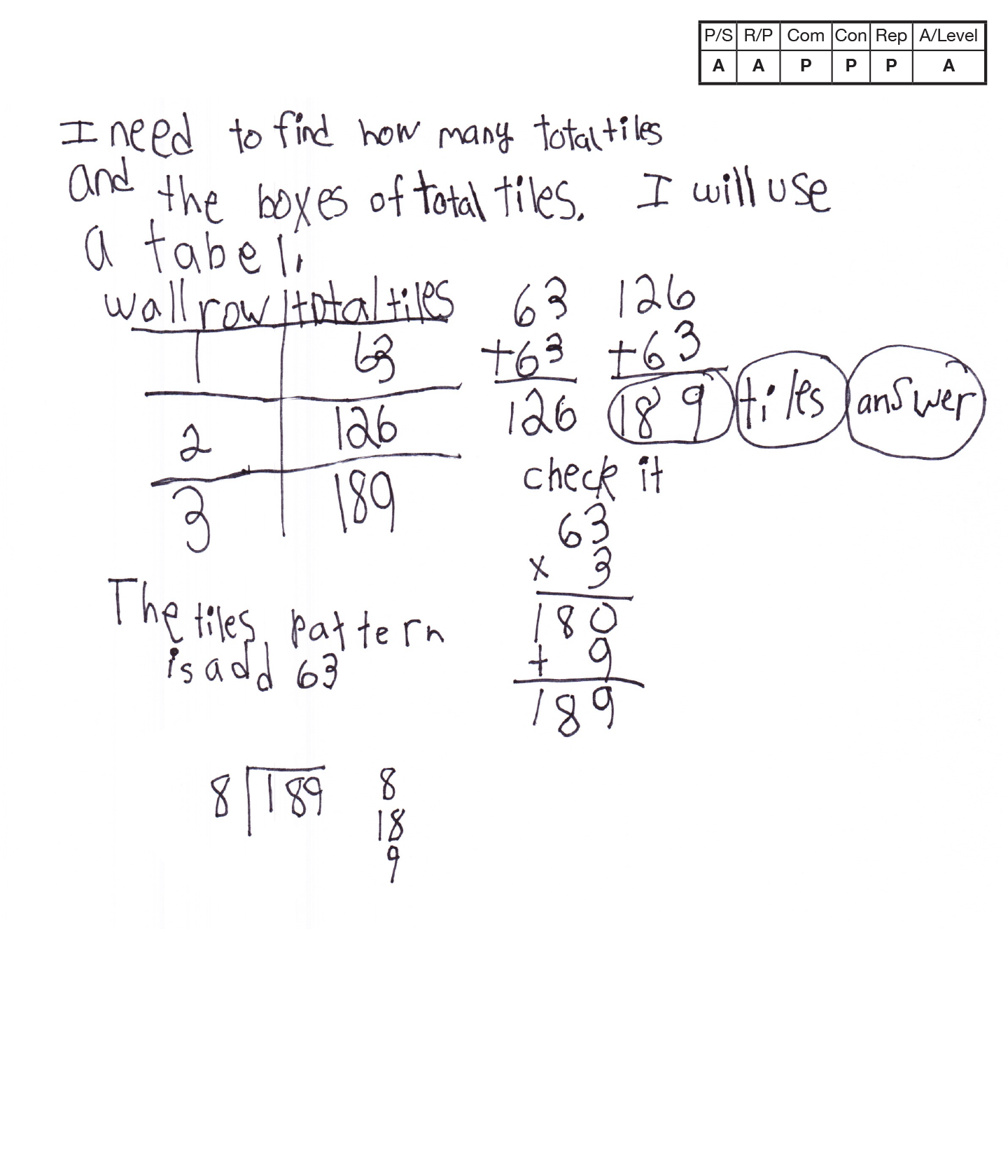This student does not meet the standard.

### Scoring Rationale

#### Apprentice

The student's strategy of using a table to find the total number of tiles Mrs. Garcia needs works to solve the first question in the task. The student's answer, "189 tiles answer" is correct. The student attempts calculation to answer the second question in the task but is not able to complete the equation.

#### Apprentice

The student demonstrates correct reasoning for the first question by using addition equations to support their table. It appears that the student understands that the second question could be solved using division but the student does not complete the calculation.

#### Practitioner

The student correctly uses the mathematical term row from the task. The student also correctly uses the terms total, "tabel," pattern.

#### Practitioner

The student makes the mathematically relevant observation, "The tiles pattern is add 63."

#### Practitioner

The student's table is appropriate and accurate. All labels are included and the data is correct.

#### Apprentice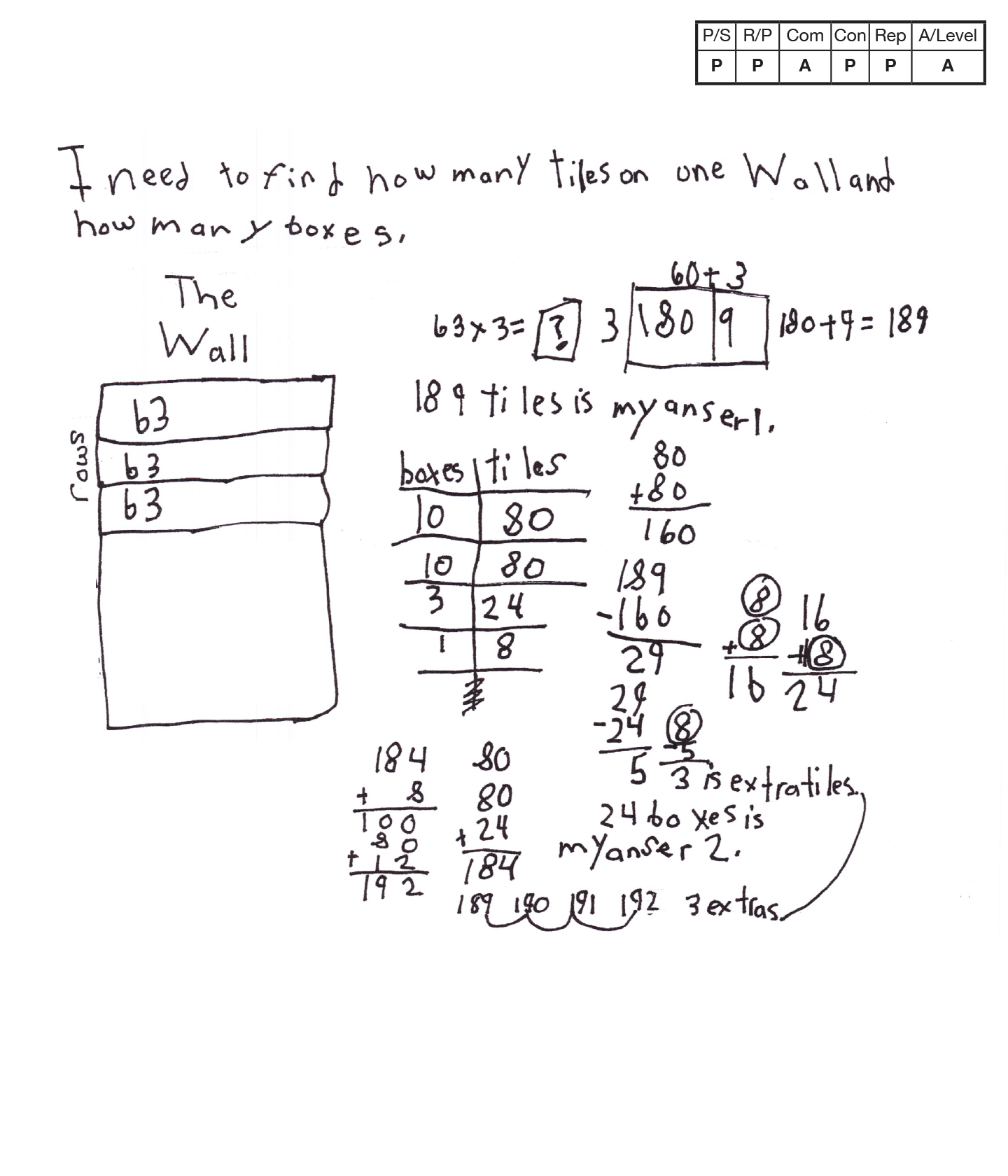This student does not meet the standard.

### Scoring Rationale

#### Practitioner

The student's strategy of using a diagram and a multiplication model to find the total number of tiles Mrs. Garcia needs for three rows works to solve the first question in the task. The student's answer, "189 tiles is my anser 1," is correct. The student's strategy of using a table to find the total number of boxes of tiles Mrs. Garcia needs works to solve the second question in the task. The student's answer, "24 boxes is my anser 2," is correct.

#### Practitioner

The student demonstrates correct reasoning of finding an unknown by using addition, subtraction, and multiplication. The student understands that adding another box of tiles is necessary to include the remainder of 5 tiles.

#### Apprentice

The student correctly uses the mathematical term row from the task.

#### Practitioner

The student makes the mathematically relevant observation, "3 is extra tiles, 3 extra."

#### Practitioner

The student's diagram is appropriate to the task and accurate. The student's table is also appropriate and accurate. All labels are included and the entered data is correct. The student also includes a multiplication model that is correct.

#### Apprentice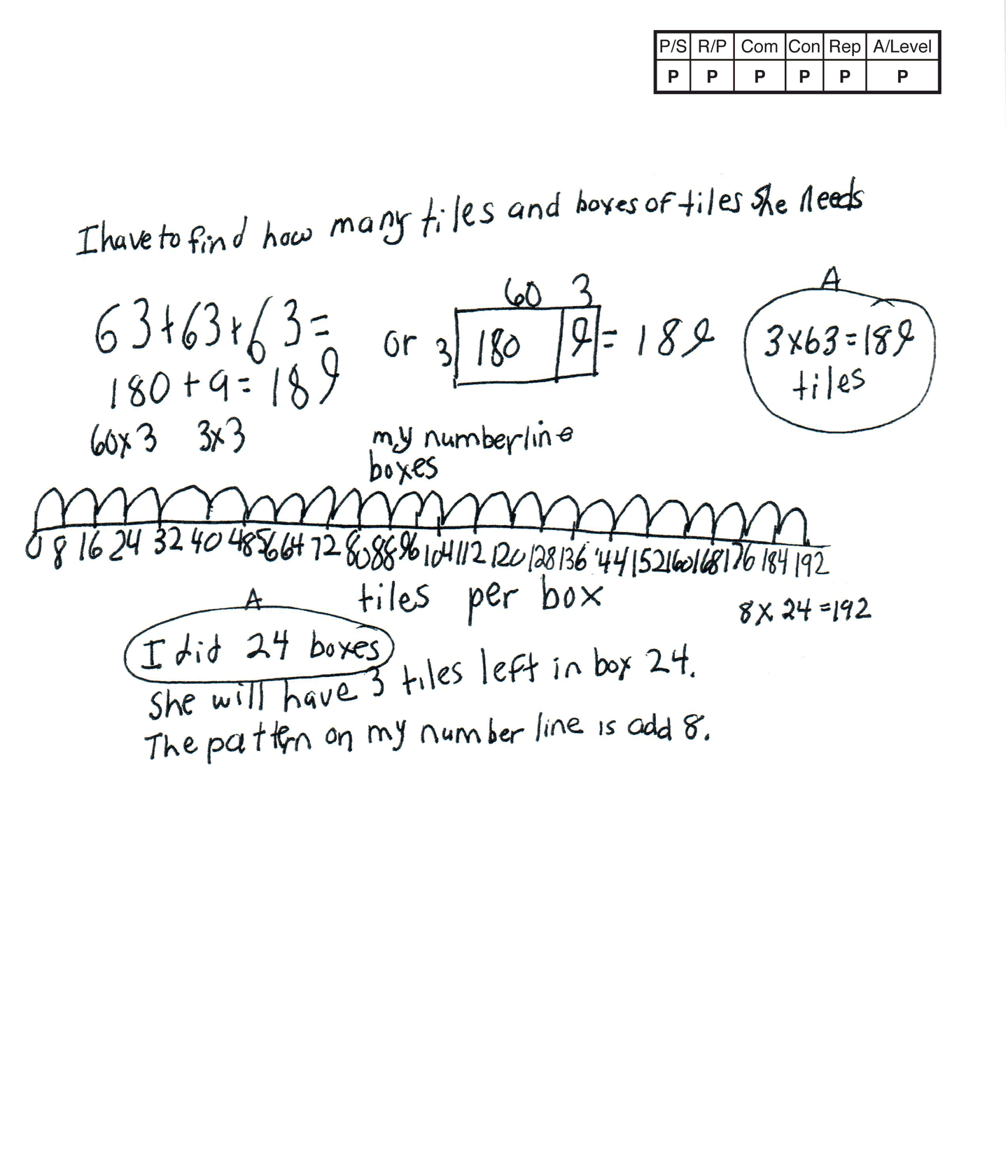This student meets the standard.

### Scoring Rationale

#### Practitioner

The student’s strategy to use multiplication to find the total number of tiles Mrs. Garcia needs for her border works to solve the task. The student’s answer, “3 x 63 =189 tiles,” is correct. The student uses a number line to find the total number of boxes of tiles Mrs. Garcia needs. The student’s answer, “I did 24 boxes” is correct.

#### Practitioner

The student demonstrates correct reasoning for the first part of the task by applying a correct multiplication equation. The student shows correct reasoning for the second part of the task by applying an add eight pattern to their number line.

#### Practitioner

The student correctly uses the mathematical terms number line, per, pattern.

#### Practitioner

The student makes the mathematically relevant observation, “She will have 3 tiles left in box 24,” and, “The pattern on my number line is add 8.”

#### Practitioner

The student’s number line is appropriate and accurate. All labels are included and the jumps for boxes are correct.

#### Practitioner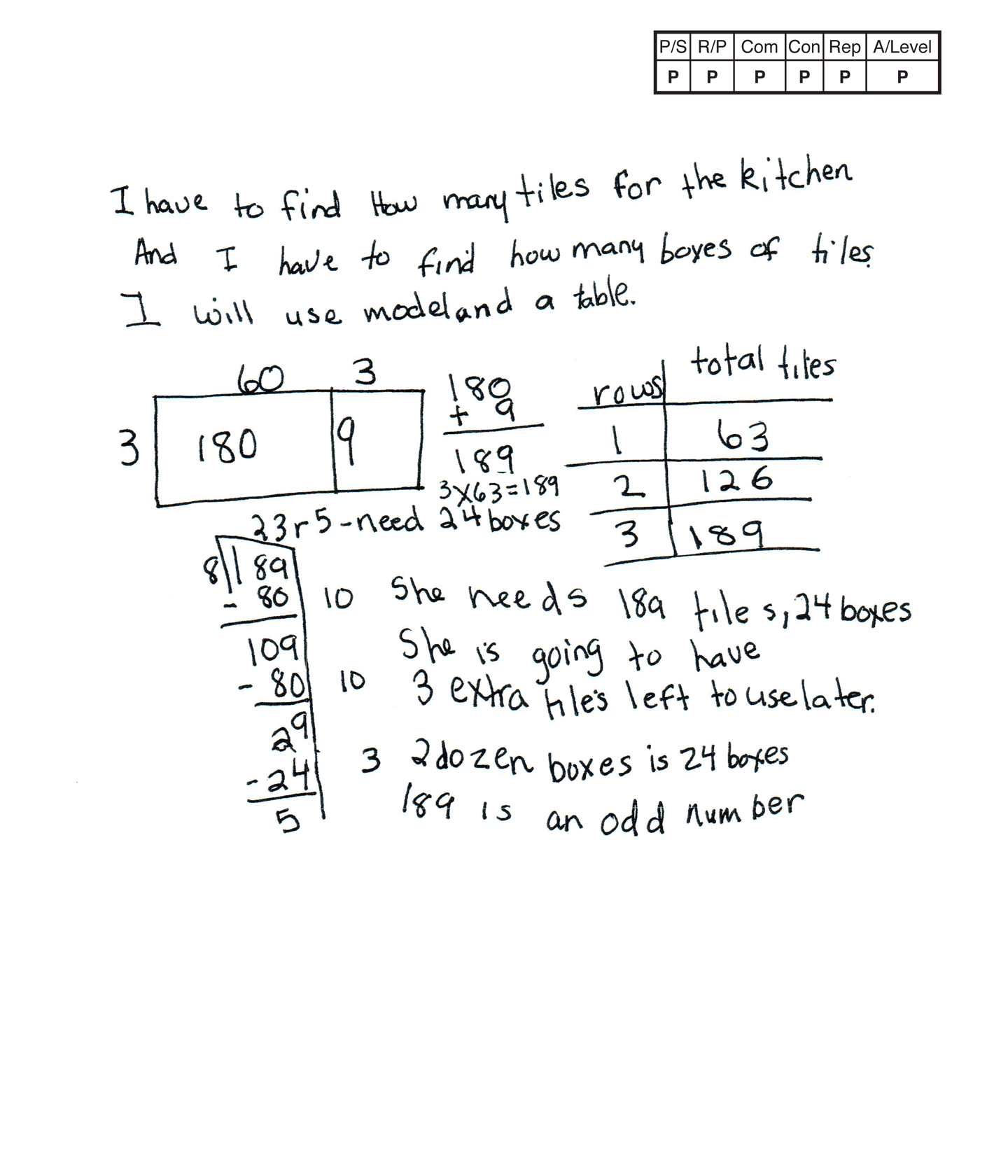This student meets the standard.

### Scoring Rationale

#### Practitioner

The student’s strategy to use a multiplication model and a table to find the total number of tiles Mrs. Garcia needs for her border works to solve the task. The student’s use of division to find the necessary number of boxes of tiles for the kitchen border works to find the answer for the second part of the task. The student’s answer, “She needs 189 tiles, 24 boxes,” is correct. The student also indicates two correct equations in their solution.

#### Practitioner

The student demonstrates correct reasoning of multiplication and division with remainder to arrive at a correct answer.

#### Practitioner

The student correctly uses the mathematical term row from the task. The student also correctly uses the terms model, table, dozen, odd number, total.

#### Practitioner

The student makes the mathematically relevant Practitioner-level observations, “She is going to have 3 extra tiles left to use later” and “2 dozen boxes is 24 boxes.” The student does not receive Practitioner-level connection credit for identifying 189 as an odd number because the student does not contiune to relate/link this statement to an underlying concept in the task.

#### Practitioner

The student’s table is appropriate and accurate. All labels are included and the entered data is correct.

#### Practitioner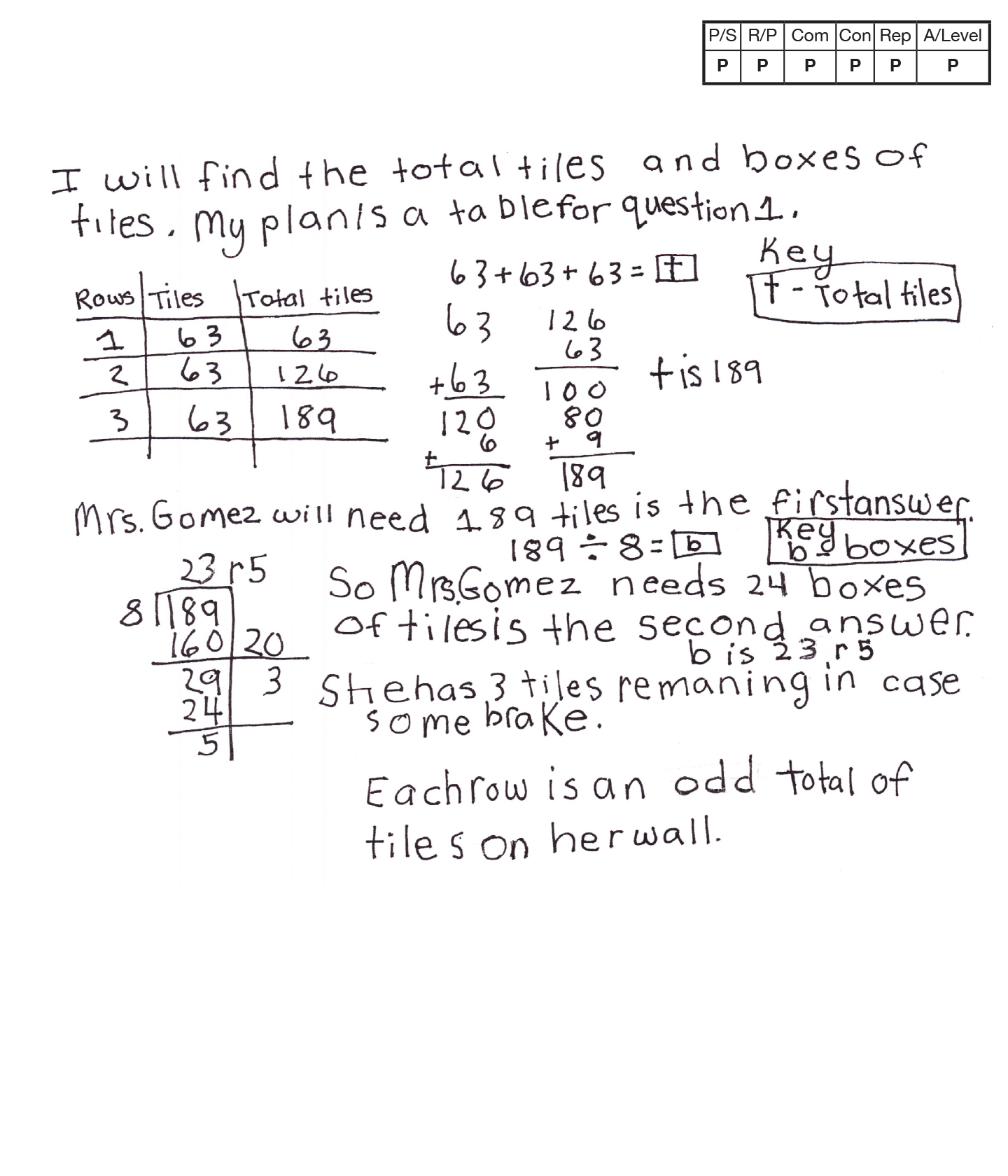This student meets the standard.

### Scoring Rationale

#### Practitioner

The student's strategy of using a table to find the total number of tiles Mrs. Garcia needs for her border works to solve the first part of the task. The student's answer, "Mrs. Gomez will need 189 tiles is the first answer," is correct. The student's use of division to find the necessary number of boxes of tiles for the kitchen border works to solve the second part of the task. The student's answer, "Mrs. Gomez needs 24 boxes of tiles is the second answer," is correct.

#### Practitioner

The student demonstrates correct reasoning of addition and division with remainder to arrive at a correct answer.

#### Practitioner

The student correctly uses the mathematical term row from the task. The student also correctly uses the terms total, table, key, first, second, odd.

#### Practitioner

The student makes the mathematically relevant observations, "She has 3 tiles remaining in case some brake," and "Each row is an odd total of tiles on her wall."

#### Practitioner

The student's table is appropriate and accurate. All labels are included and the entered data is correct.

#### Practitioner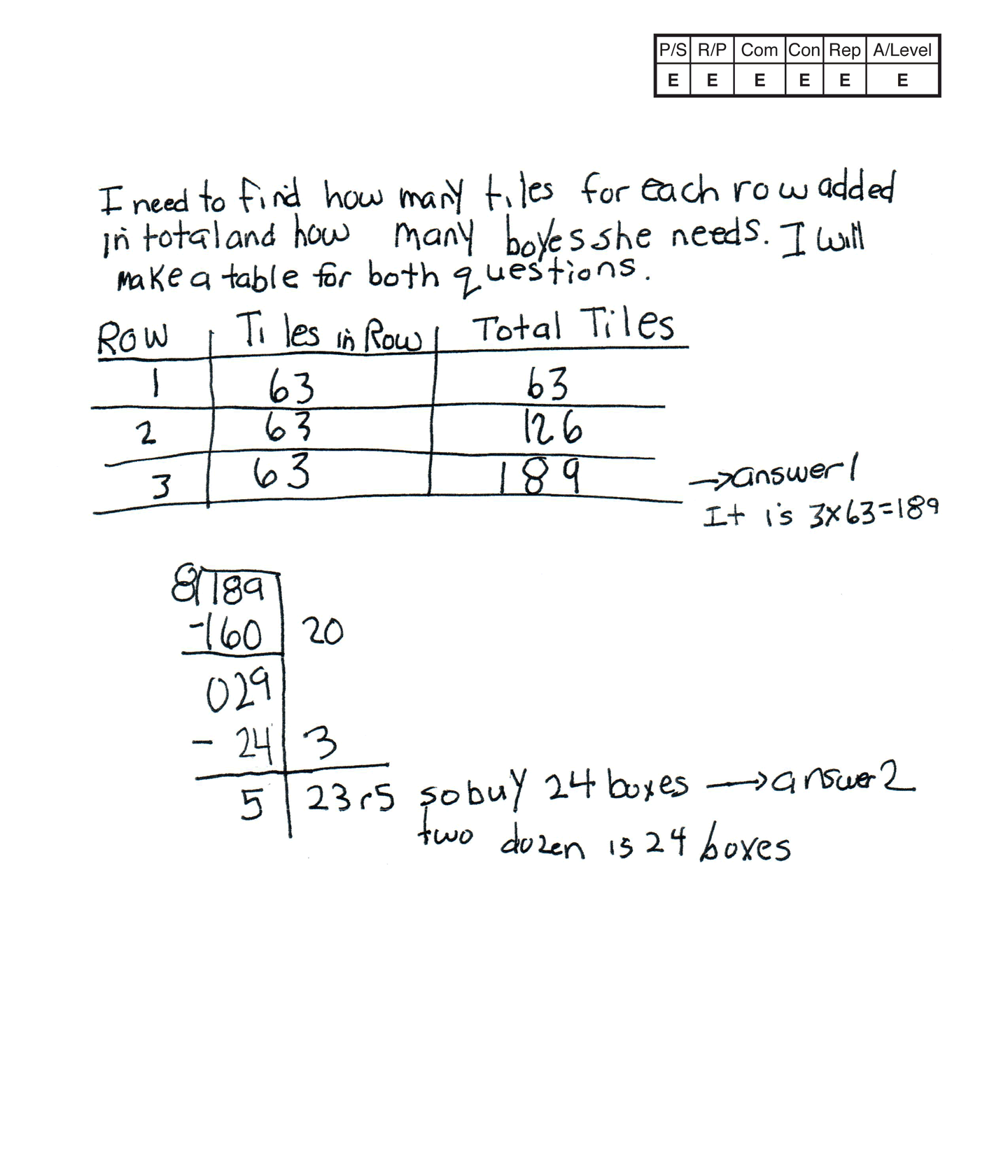,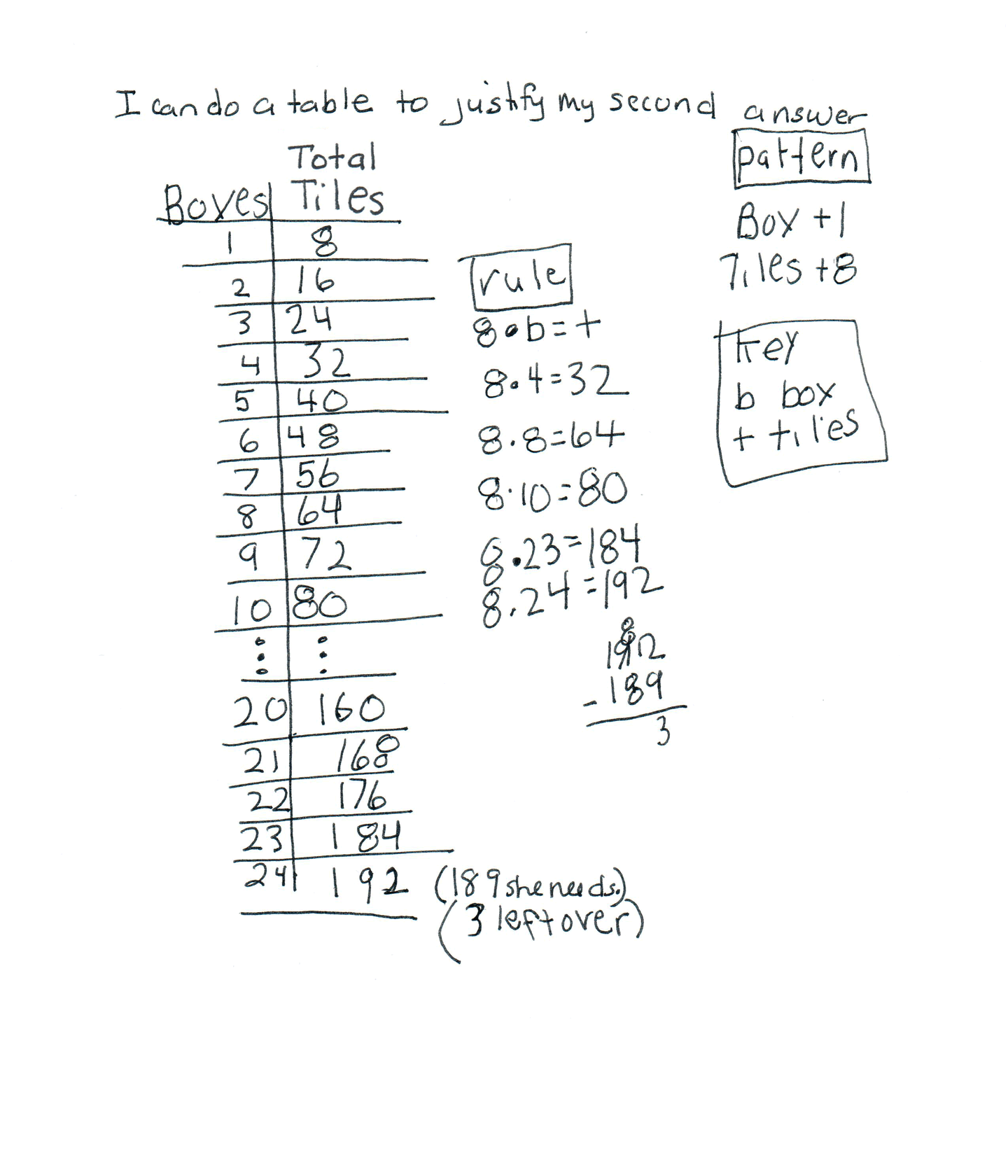This student exceeds the standard.

### Scoring Rationale

#### Expert

The student’s strategy to use a table and multiplication to find the total number of tiles Mrs. Garcia needs for her border works to solve the task. The student’s answer, “3 x 63 = 189 tiles,” is correct. The student uses division to find the total number of boxes of tiles Mrs. Garcia needs. The student’s answer, “So buy 24 boxes” is correct. The student uses alternate strategies of a table and generalized rule.

#### Expert

The student demonstrates correct reasoning of multiplication and division as well as generalizing and applying a rule, 8 · b = t, to justify the second part of the task.

#### Expert

The student correctly uses the mathematical term row from the task. The student also correctly uses the terms total, table, dozen, second, pattern, key, rule. The student correctly uses the mathematical symbolic notation 8 · b = t.

#### Expert

The student makes the mathematically relevant Practitioner observations, “two dozen is 24 boxes,” and, “3 left over.” The student makes an Expert connection. The student generalizes a rule, 8 · b = t, and applies the rule for 4, 8, 10, 23, and 24 boxes. The student defines the variables b and t in a key.

#### Expert

The student’s tables are appropriate to the task and are accurate. All columns are labeled correctly and the entered data is correct. The student creates the second table to justify their division equation and to extend their thinking to a generalized rule.